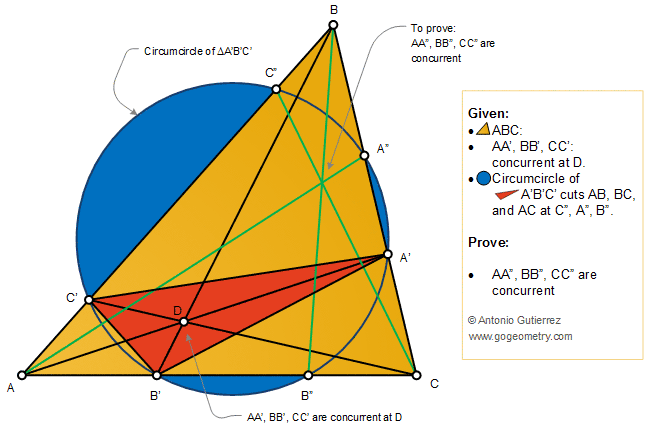# Online Geometry Problem 488: Triangle, Cevian, Concurrency, Circle, Circumcircle

 The figure shows a triangle ABC with the concurrent cevians AA', BB', and CC'. The circumcircle of triangle A'B'C' cuts AB, BC, and AC at C", A", and B", respectively. Prove that the cevians AA", BB", and CC" are concurrent. Circumcircle of a triangle is the circle which passes through the vertices. A cevian is a segment which joins a vertex of a triangle with a point on the opposite side (or its extension).Home | Search | Geometry | Problems | All Problems | 481-490 | Email | Solution / comment | By Antonio Gutierrez